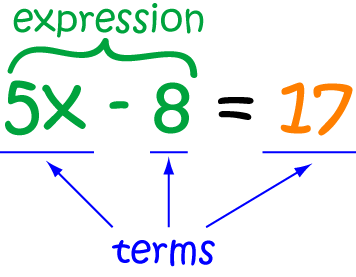Reading:

# Definition of EquationAn equation is a mathematical expression that tells us that two things are equal.

Equations always include the equals sign, "$=$"

Here are some examples of equations:

• $2 \times 3 = 7 - 1$. This says that $2 \times 3$ and $7 - 1$ are equal to each other. They are! They are both equal to $6$.
• $3x + 3 = 9$ is another equation. It says that $3x + 3$ has to be the same as $9$.

### Description

The aim of this dictionary is to provide definitions to common mathematical terms. Students learn a new math skill every week at school, sometimes just before they start a new skill, if they want to look at what a specific term means, this is where this dictionary will become handy and a go-to guide for a student.

### Audience

Year 1 to Year 12 students

### Learning Objectives

Learn common math terms starting with letter E

Author: Subject Coach
Added on: 6th Feb 2018

You must be logged in as Student to ask a Question.

None just yet!